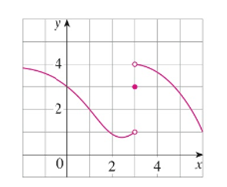Chapter 2.2, Problem 5E

Chapter
Section
Textbook Problem

For the function f whose graph is given, state the value of each quantity, if it exists. If it does not exit, explain why.(a) lim x → 1 f ( x ) (b) lim x → 3 − f ( x ) (c) lim x → 3 + f ( x ) (d) lim x → 3 f ( x ) (e) f ( 3 )(a)

To determine

To find: The value of limx1f(x) if exists.

Explanation

From the given graph, it is found that the curve move towards y=2 as x approaches 1 from the left, that is x<1.

That is, limx1f(x)=2.

From the given graph, it is found that the curve move towards y=2 as x approaches 1 from the right, that is x>1.

That is, limx1+f(x)=2

(b)

To determine

To find: The value of limx3f(x) if exists.

(c)

To determine

To find: The value of limx3+f(x) if exists.

(d)

To determine

To find: The value of limx3f(x) if exists.

(e)

To determine

To find: The value of f(3).

Still sussing out bartleby?

Check out a sample textbook solution.

See a sample solution

The Solution to Your Study Problems

Bartleby provides explanations to thousands of textbook problems written by our experts, many with advanced degrees!

Get Started

In Problem 8-10, solve the inequality and graph the solution

Mathematical Applications for the Management, Life, and Social Sciences

True or False: Du f(a, b) = ∇(a, b) · u.

Study Guide for Stewart's Multivariable Calculus, 8th

Define and compute the three measures of central tendency.

Research Methods for the Behavioral Sciences (MindTap Course List)